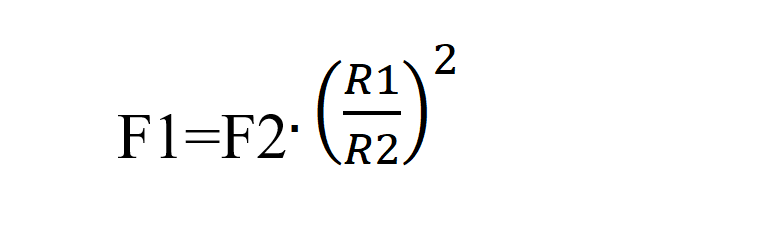# Fa-6 Hydraulic Press Syringes

## Aim

To demonstrate that mechanical advantage can be obtained from utilising the pressure applied to a fluid.

## Apparatus

• 4 Syringes of Different Diameter
• Silicon Tubing
• WaterHydraulic Press Syringes Apparatus

## Description

The equation of pressure being applied to a fluid is given

by the equationWhen we have a 2 Syringe system

The equation appliesWhere F1 is the Force applied to syringe 1 with an area A1 with radius R1

F2 is the Force applied to syringe 2 with an area A2 with radius R2

The area of a syringe is given by the equation A=πr2

The equation can be rewrittenDepending on the ratio of the radii of the syringes, the force applied to one

syringe can greatly magnify the resultant force applied to second syringe.